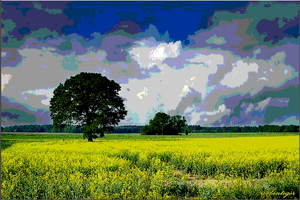```kernel Snn { parameter float size < minValue: 0.0; maxValue: 8.0; defaultValue: 2.0; >; parameter int step < minValue: 1; maxValue: 10; defaultValue: 1; >; void evaluatePixel(in image4 src, out pixel4 result) { pixel2 coord = outCoord(); pixel4 valueC = sampleLinear(src, coord); float theStep = float(step); float2 point = float2(0.0,0.0); float x = 0.0; float y = 0.0; float count = 0.0; pixel4 sum = pixel4(0.0, 0.0, 0.0, 0.0); pixel4 valueA; pixel4 valueB; for( x = - size; x < size; x+= theStep) { for(y = -size; y < size; y+= theStep) { valueA = sampleLinear(src, pixel2(coord.x + x, coord.y + y)); valueB = sampleLinear(src, pixel2(coord.x - x, coord.y - y)); if ( any(lessThan(abs(valueC - valueA), abs(valueC - valueB)))) { sum = sum + valueA; } else { sum = sum + valueB; } count = count + 1.0; } } result = sum/count; } }``````kernel Websafe { parameter float value < minValue: 0.0; maxValue: 500.0; defaultValue: 10.0; >; void evaluatePixel( in image4 src, out pixel4 result) { result = sampleLinear(src, outCoord()); result = (floor((result*255.0)/51.0) * 51.0)/255.0; } }```